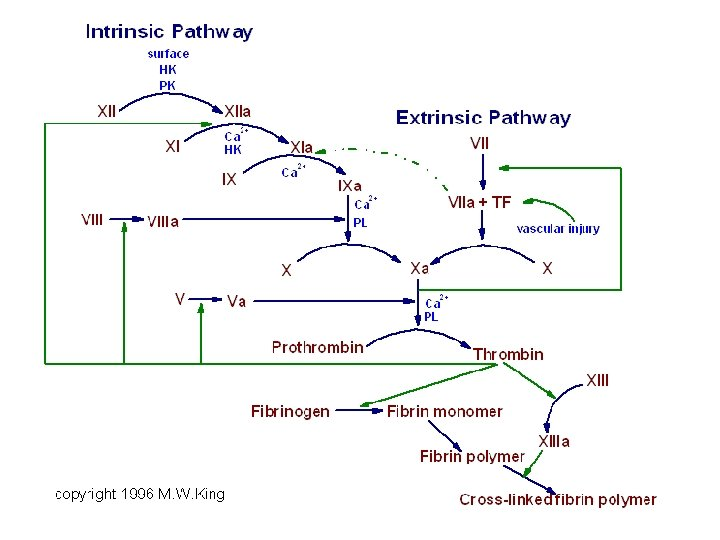Скачать презентацию Coordination Chemistry Transition elements partly filed d or

5b758037f958a869057777a032d4e25e.ppt

• Количество слайдов: 29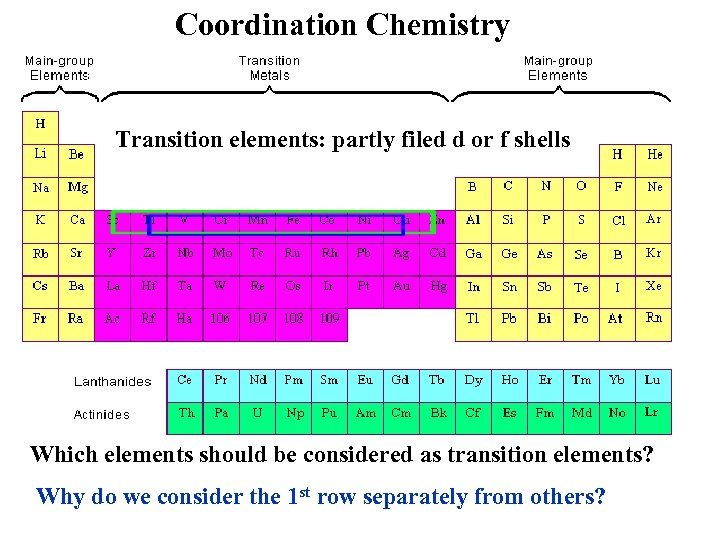Coordination Chemistry Transition elements: partly filed d or f shells Which elements should be considered as transition elements? Why do we consider the 1 st row separately from others?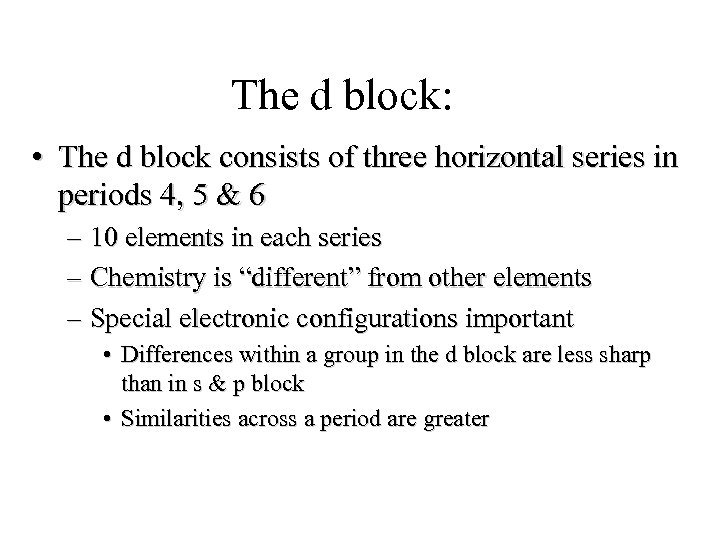The d block: • The d block consists of three horizontal series in periods 4, 5 & 6 – 10 elements in each series – Chemistry is “different” from other elements – Special electronic configurations important • Differences within a group in the d block are less sharp than in s & p block • Similarities across a period are greater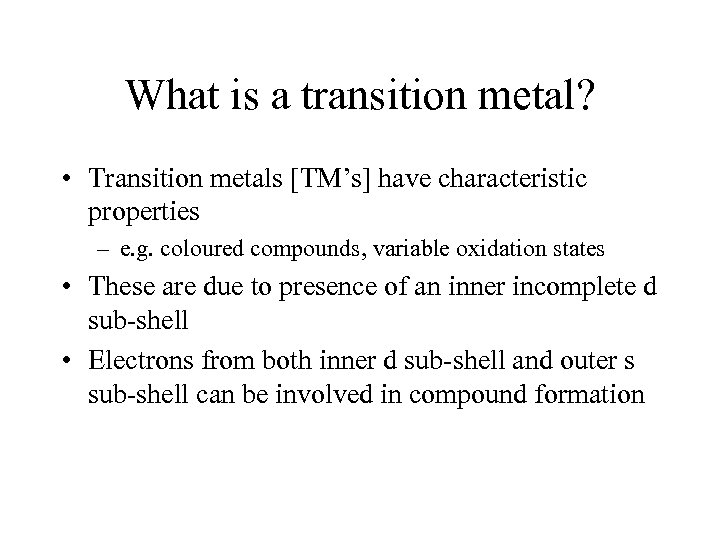What is a transition metal? • Transition metals [TM’s] have characteristic properties – e. g. coloured compounds, variable oxidation states • These are due to presence of an inner incomplete d sub-shell • Electrons from both inner d sub-shell and outer s sub-shell can be involved in compound formation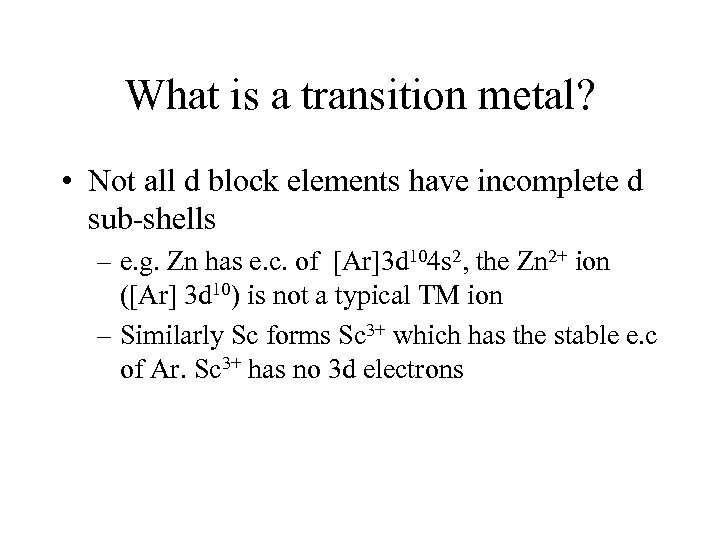What is a transition metal? • Not all d block elements have incomplete d sub-shells – e. g. Zn has e. c. of [Ar]3 d 104 s 2, the Zn 2+ ion ([Ar] 3 d 10) is not a typical TM ion – Similarly Sc forms Sc 3+ which has the stable e. c of Ar. Sc 3+ has no 3 d electrons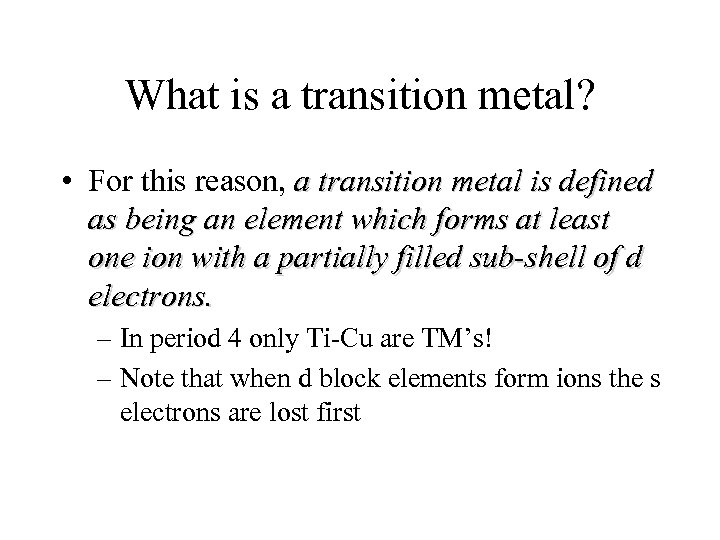What is a transition metal? • For this reason, a transition metal is defined as being an element which forms at least one ion with a partially filled sub-shell of d electrons. – In period 4 only Ti-Cu are TM’s! – Note that when d block elements form ions the s electrons are lost first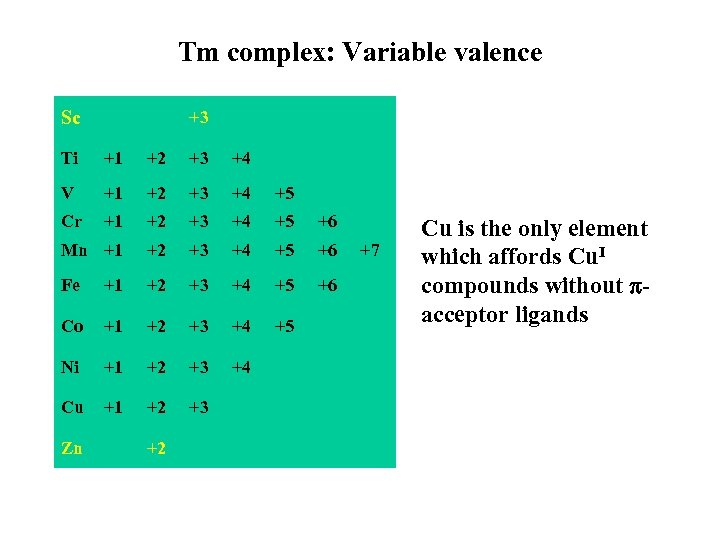Tm complex: Variable valence Sc +3 Ti +1 +2 +3 +4 V +1 +2 +3 +4 +5 Cr +1 +2 +3 +4 +5 +6 Mn +1 +2 +3 +4 +5 +6 Fe +1 +2 +3 +4 +5 +6 Co +1 +2 +3 +4 +5 Ni +1 +2 +3 +4 Cu +1 +2 +3 Zn +2 +7 Cu is the only element which affords Cu. I compounds without acceptor ligands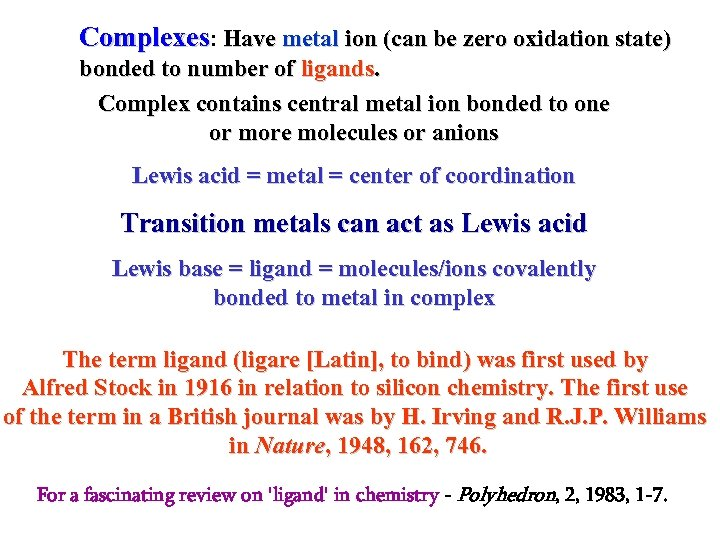Complexes: Have metal ion (can be zero oxidation state) bonded to number of ligands. Complex contains central metal ion bonded to one or more molecules or anions Lewis acid = metal = center of coordination Transition metals can act as Lewis acid Lewis base = ligand = molecules/ions covalently bonded to metal in complex The term ligand (ligare [Latin], to bind) was first used by Alfred Stock in 1916 in relation to silicon chemistry. The first use of the term in a British journal was by H. Irving and R. J. P. Williams in Nature, 1948, 162, 746. For a fascinating review on 'ligand' in chemistry - Polyhedron, 2, 1983, 1 -7.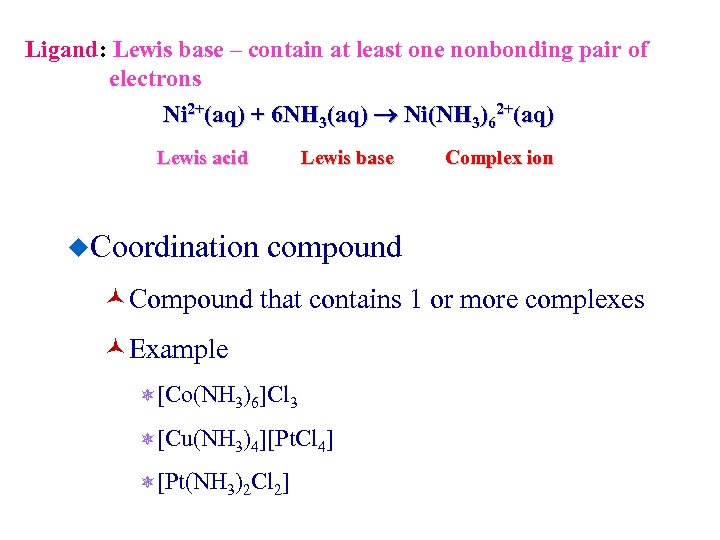Ligand: Lewis base – contain at least one nonbonding pair of electrons Ni 2+(aq) + 6 NH 3(aq) Ni(NH 3)62+(aq) Lewis acid Lewis base Complex ion ¿Coordination compound ©Compound that contains 1 or more complexes ©Example ô[Co(NH 3)6]Cl 3 ô[Cu(NH 3)4][Pt. Cl 4] ô[Pt(NH 3)2 Cl 2]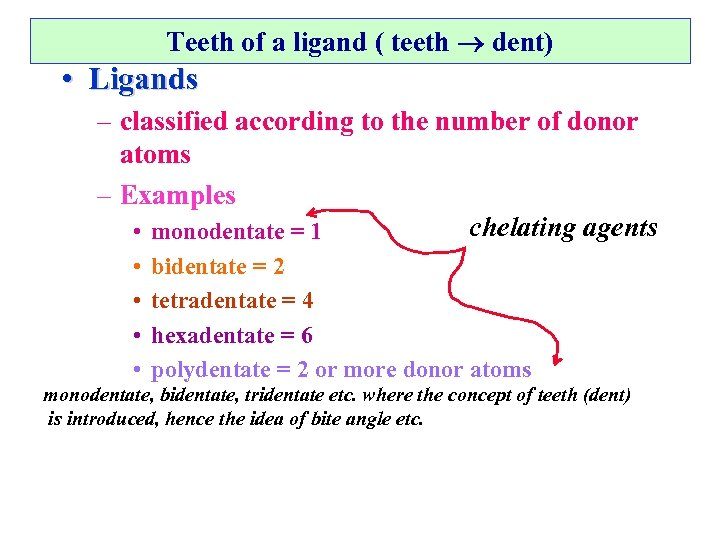Teeth of a ligand ( teeth dent) • Ligands – classified according to the number of donor atoms – Examples chelating agents • monodentate = 1 • • bidentate = 2 tetradentate = 4 hexadentate = 6 polydentate = 2 or more donor atoms monodentate, bidentate, tridentate etc. where the concept of teeth (dent) is introduced, hence the idea of bite angle etc.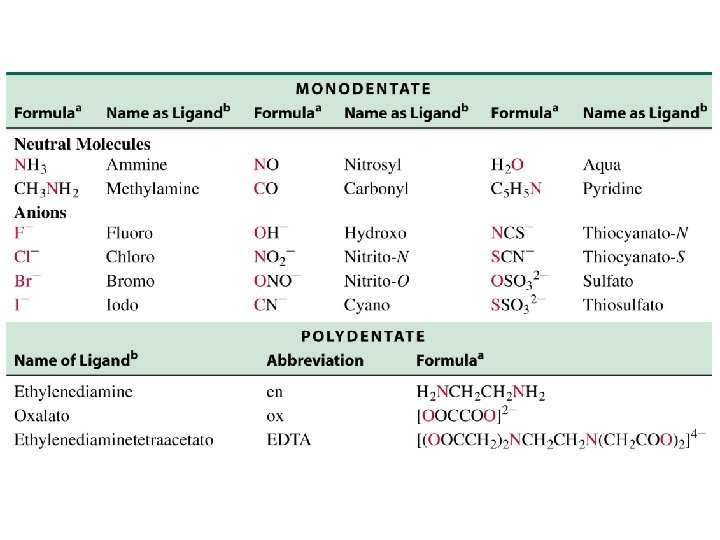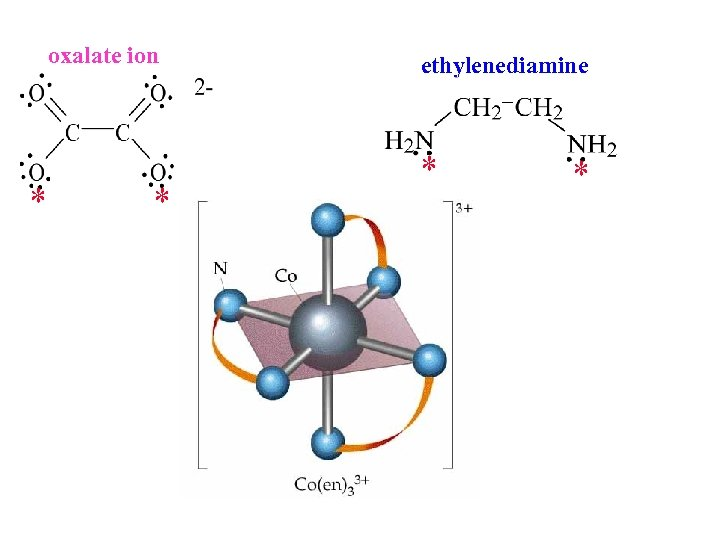oxalate ion * * ethylenediamine * *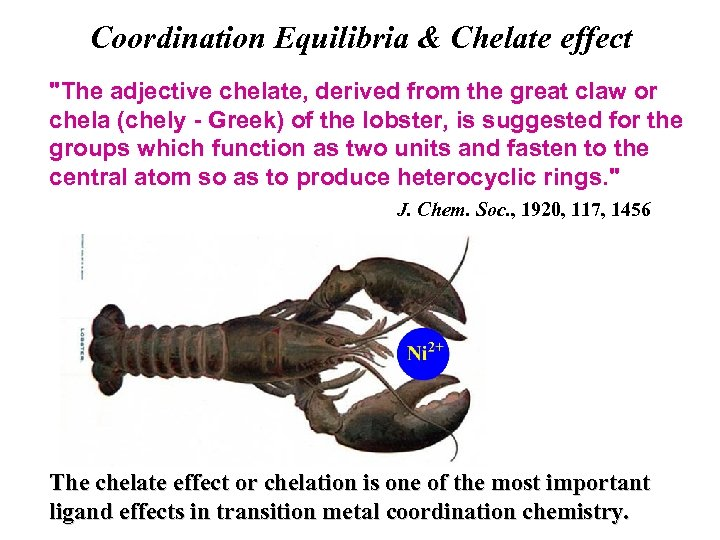Coordination Equilibria & Chelate effect "The adjective chelate, derived from the great claw or chela (chely - Greek) of the lobster, is suggested for the groups which function as two units and fasten to the central atom so as to produce heterocyclic rings. " J. Chem. Soc. , 1920, 117, 1456 The chelate effect or chelation is one of the most important ligand effects in transition metal coordination chemistry.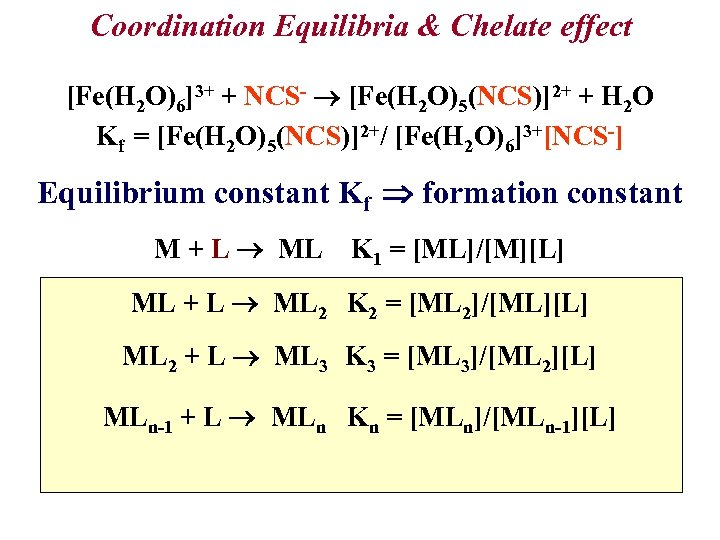Coordination Equilibria & Chelate effect [Fe(H 2 O)6]3+ + NCS- [Fe(H 2 O)5(NCS)]2+ + H 2 O Kf = [Fe(H 2 O)5(NCS)]2+/ [Fe(H 2 O)6]3+[NCS-] Equilibrium constant Kf formation constant M + L ML K 1 = [ML]/[M][L] ML + L ML 2 K 2 = [ML 2]/[ML][L] ML 2 + L ML 3 K 3 = [ML 3]/[ML 2][L] MLn-1 + L MLn Kn = [MLn]/[MLn-1][L]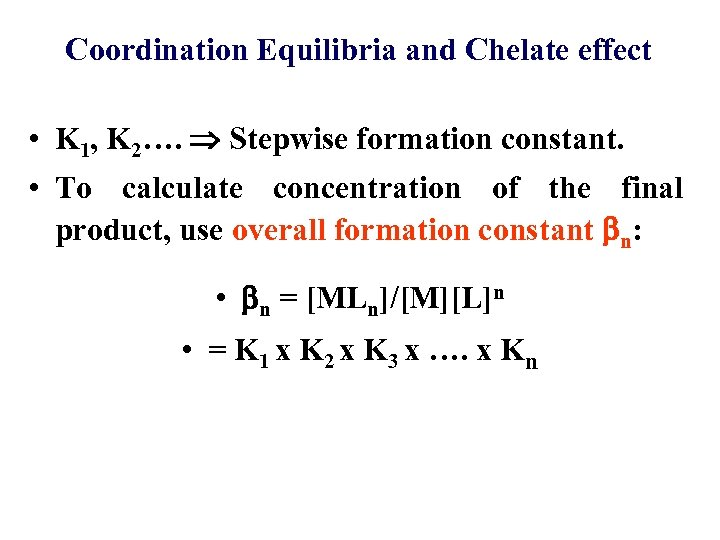Coordination Equilibria and Chelate effect • K 1, K 2…. Stepwise formation constant. • To calculate concentration of the final product, use overall formation constant n: • n = [MLn]/[M][L]n • = K 1 x K 2 x K 3 x …. x Kn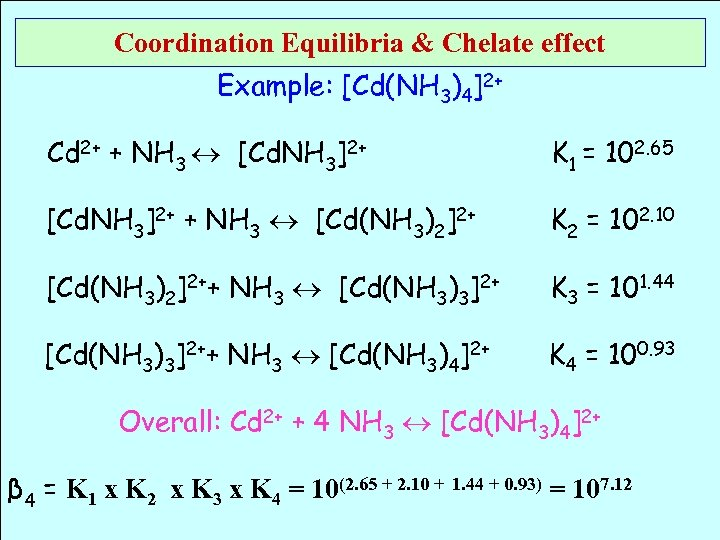Coordination Equilibria & Chelate effect Example: [Cd(NH 3)4]2+ Cd 2+ + NH 3 [Cd. NH 3]2+ K 1 = 102. 65 [Cd. NH 3]2+ + NH 3 [Cd(NH 3)2]2+ K 2 = 102. 10 [Cd(NH 3)2]2++ NH 3 [Cd(NH 3)3]2+ K 3 = 101. 44 [Cd(NH 3)3]2++ NH 3 [Cd(NH 3)4]2+ K 4 = 100. 93 Overall: Cd 2+ + 4 NH 3 [Cd(NH 3)4]2+ β 4 = K 1 x K 2 x K 3 x K 4 = 10(2. 65 + 2. 10 + 1. 44 + 0. 93) = 107. 12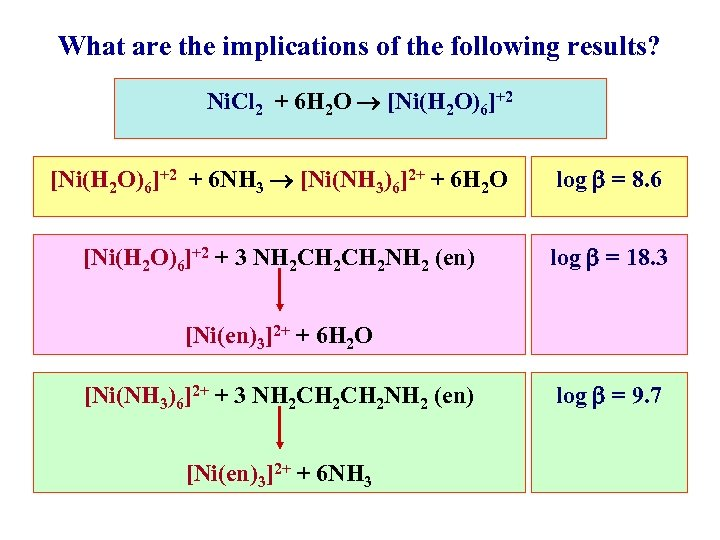What are the implications of the following results? Ni. Cl 2 + 6 H 2 O [Ni(H 2 O)6]+2 + 6 NH 3 [Ni(NH 3)6]2+ + 6 H 2 O log = 8. 6 [Ni(H 2 O)6]+2 + 3 NH 2 CH 2 NH 2 (en) log = 18. 3 [Ni(en)3]2+ + 6 H 2 O [Ni(NH 3)6]2+ + 3 NH 2 CH 2 NH 2 (en) [Ni(en)3]2+ + 6 NH 3 log = 9. 7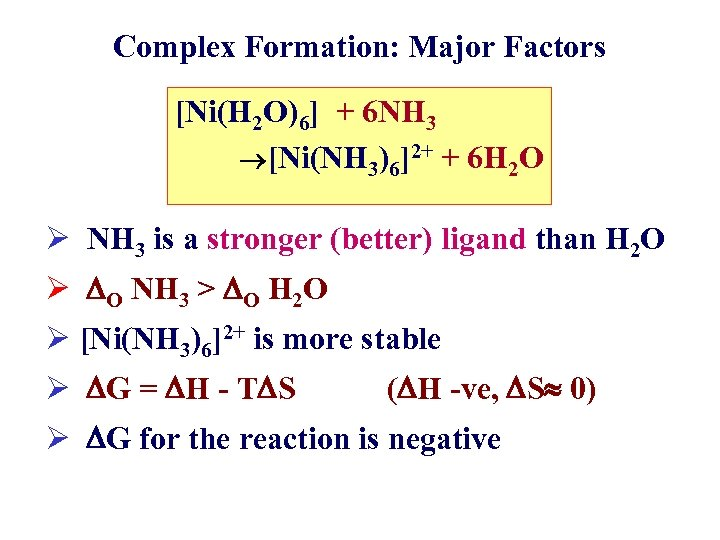Complex Formation: Major Factors [Ni(H 2 O)6] + 6 NH 3 [Ni(NH 3)6]2+ + 6 H 2 O Ø NH 3 is a stronger (better) ligand than H 2 O Ø O NH 3 > O H 2 O Ø [Ni(NH 3)6]2+ is more stable Ø G = H - T S ( H -ve, S 0) Ø G for the reaction is negative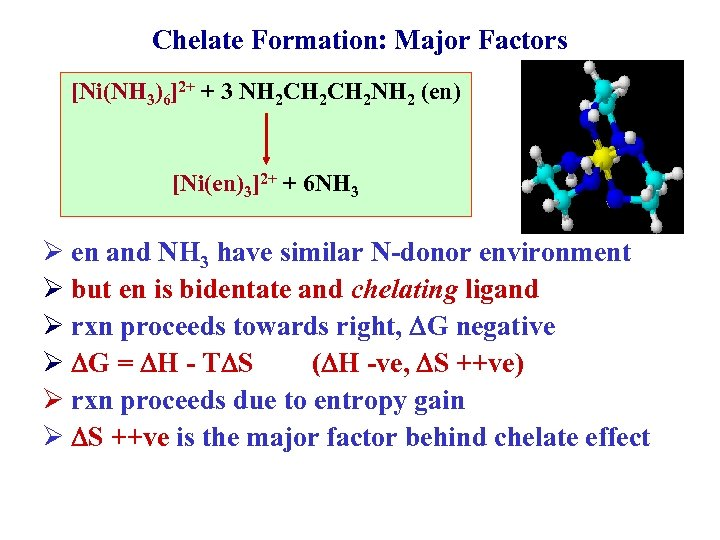Chelate Formation: Major Factors [Ni(NH 3)6]2+ + 3 NH 2 CH 2 NH 2 (en) [Ni(en)3]2+ + 6 NH 3 Ø en and NH 3 have similar N-donor environment Ø but en is bidentate and chelating ligand Ø rxn proceeds towards right, G negative Ø G = H - T S ( H -ve, S ++ve) Ø rxn proceeds due to entropy gain Ø S ++ve is the major factor behind chelate effect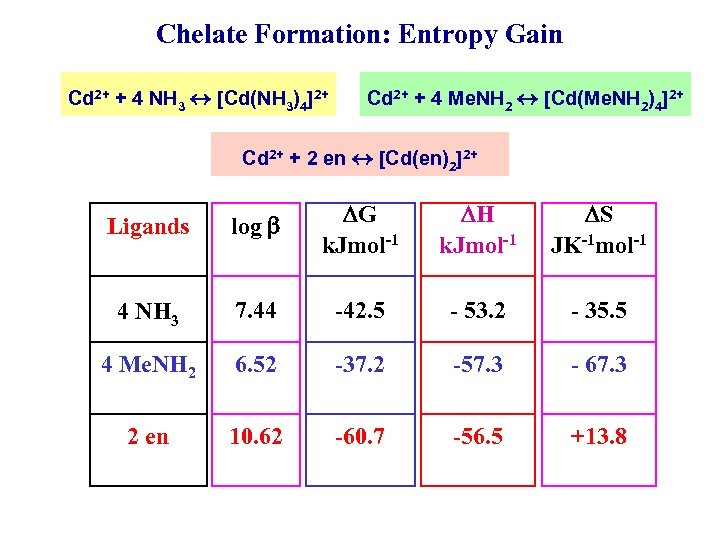Chelate Formation: Entropy Gain Cd 2+ + 4 NH 3 [Cd(NH 3)4]2+ Cd 2+ + 4 Me. NH 2 [Cd(Me. NH 2)4]2+ Cd 2+ + 2 en [Cd(en)2]2+ Ligands log G k. Jmol-1 H k. Jmol-1 S JK-1 mol-1 4 NH 3 7. 44 -42. 5 - 53. 2 - 35. 5 4 Me. NH 2 6. 52 -37. 2 -57. 3 - 67. 3 2 en 10. 62 -60. 7 -56. 5 +13. 8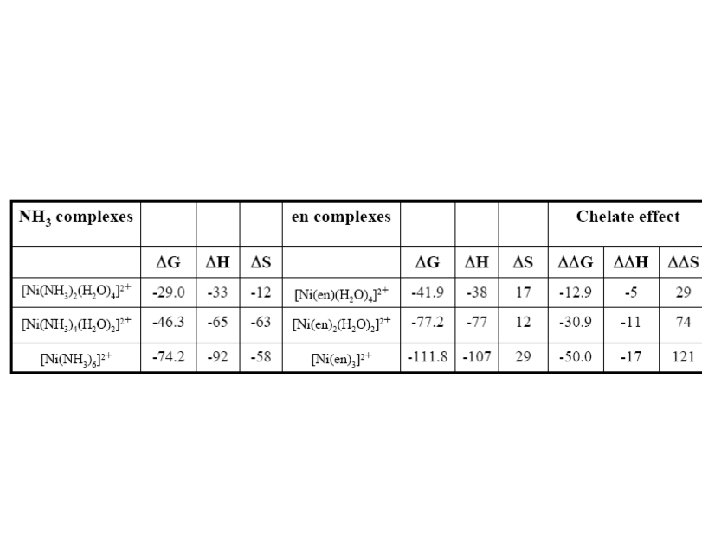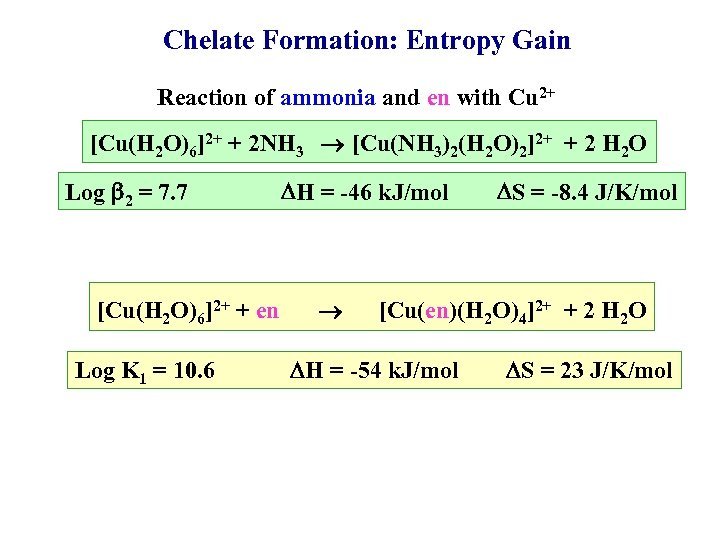Chelate Formation: Entropy Gain Reaction of ammonia and en with Cu 2+ [Cu(H 2 O)6]2+ + 2 NH 3 [Cu(NH 3)2(H 2 O)2]2+ + 2 H 2 O Log 2 = 7. 7 [Cu(H 2 O)6]2+ + en Log K 1 = 10. 6 H = -46 k. J/mol S = -8. 4 J/K/mol [Cu(en)(H 2 O)4]2+ + 2 H 2 O H = -54 k. J/mol S = 23 J/K/mol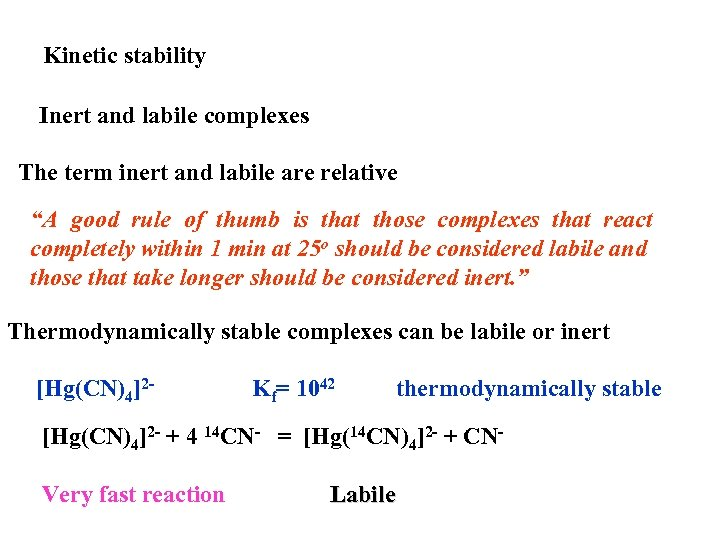Kinetic stability Inert and labile complexes The term inert and labile are relative “A good rule of thumb is that those complexes that react completely within 1 min at 25 o should be considered labile and those that take longer should be considered inert. ” Thermodynamically stable complexes can be labile or inert [Hg(CN)4]2 - Kf= 1042 thermodynamically stable [Hg(CN)4]2 - + 4 14 CN- = [Hg(14 CN)4]2 - + CNVery fast reaction Labile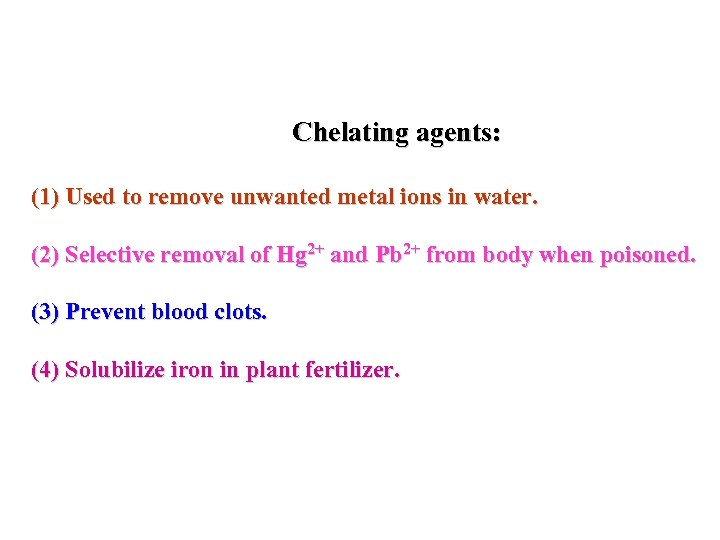Chelating agents: (1) Used to remove unwanted metal ions in water. (2) Selective removal of Hg 2+ and Pb 2+ from body when poisoned. (3) Prevent blood clots. (4) Solubilize iron in plant fertilizer.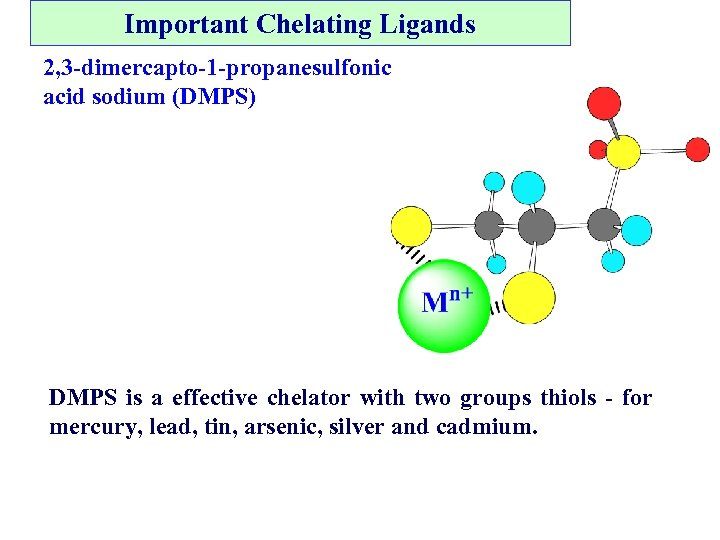Important Chelating Ligands 2, 3 -dimercapto-1 -propanesulfonic acid sodium (DMPS) DMPS is a effective chelator with two groups thiols - for mercury, lead, tin, arsenic, silver and cadmium.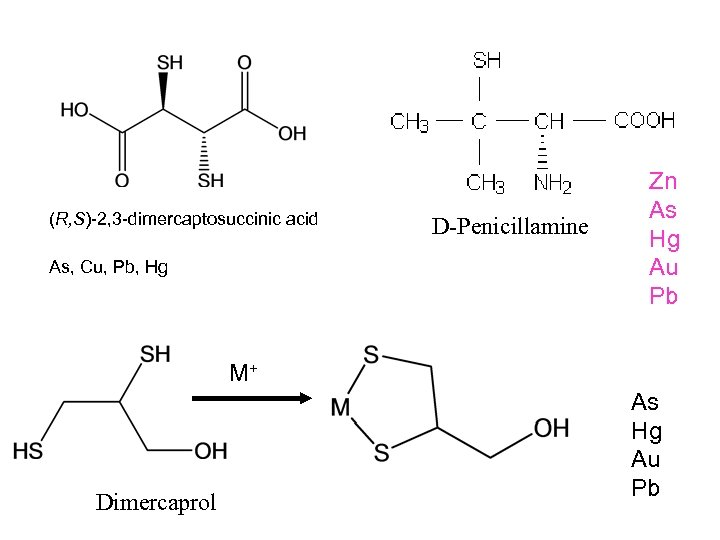(R, S)-2, 3 -dimercaptosuccinic acid As, Cu, Pb, Hg D-Penicillamine Zn As Hg Au Pb M+ Dimercaprol As Hg Au Pb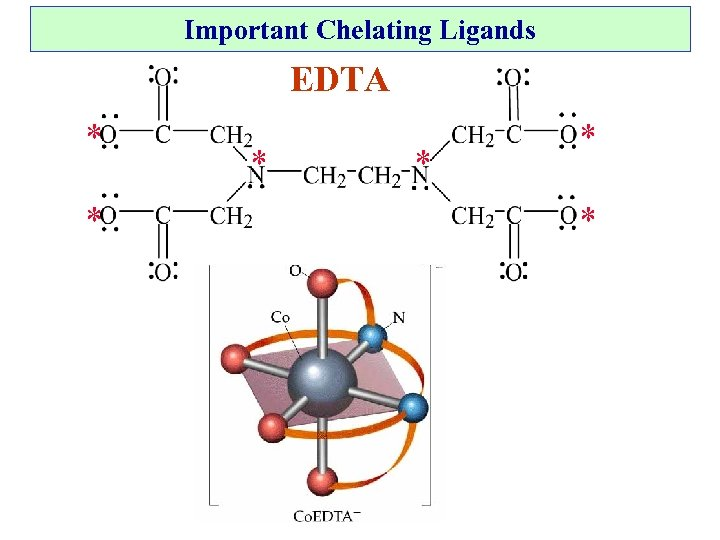Important Chelating Ligands EDTA * * *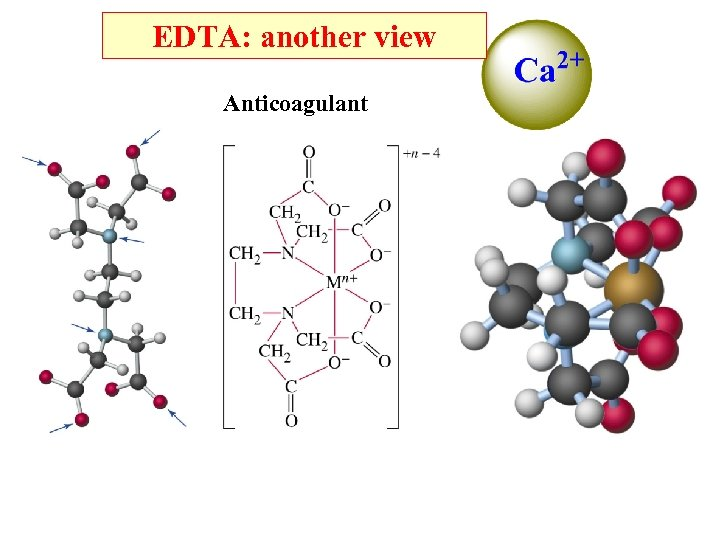EDTA: another view Anticoagulant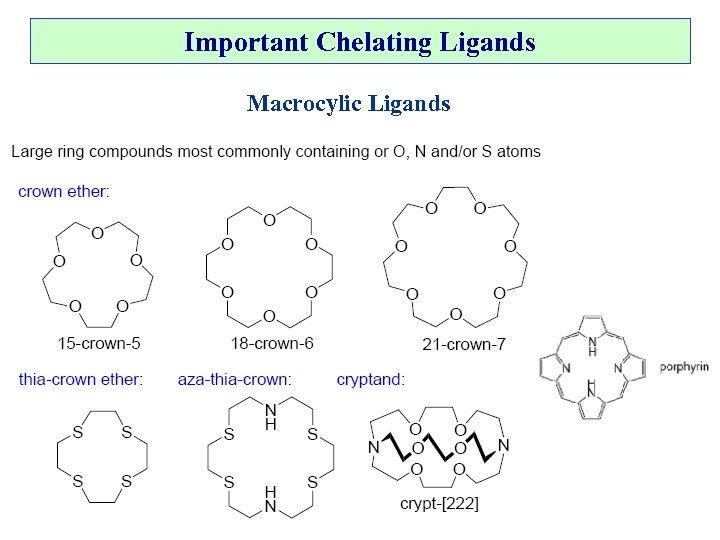Important Chelating Ligands Macrocylic Ligands